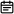# 88. 合并两个有序数组2019-07-08

## 题目链接

https://leetcode-cn.com/problems/merge-sorted-array/

## 题目描述

• 初始化 `nums1``nums2` 的元素数量分别为 `m``n`
• 你可以假设 `nums1` 有足够的空间（空间大小大于或等于 `m + n`）来保存 `nums2` 中的元素。

``````输入:
nums1 = [1,2,3,0,0,0], m = 3
nums2 = [2,5,6],       n = 3

## 解题方案

### 思路

• 标签：从后向前数组遍历
• 因为`nums1`的空间都集中在后面，所以从后向前处理排序的数据会更好，节省空间，一边遍历一边将值填充进去
• 设置指针`len1``len2`分别指向`nums1``nums2`的有数字尾部，从尾部值开始比较遍历，同时设置指针`len`指向`nums1`的最末尾，每次遍历比较值大小之后，则进行填充
• `len1<0`时遍历结束，此时`nums2`中海油数据未拷贝完全，将其直接拷贝到`nums1`的前面，最后得到结果数组
• 时间复杂度：O(m+n)

### 代码

• Java版本
``````class Solution {
public void merge(int[] nums1, int m, int[] nums2, int n) {
int len1 = m - 1;
int len2 = n - 1;
int len = m + n - 1;
while(len1 >= 0 && len2 >= 0) {
// 注意--符号在后面，表示先进行计算再减1，这种缩写缩短了代码
nums1[len--] = nums1[len1] > nums2[len2] ? nums1[len1--] : nums2[len2--];
}
// 表示将nums2数组从下标0位置开始，拷贝到nums1数组中，从下标0位置开始，长度为len2+1
System.arraycopy(nums2, 0, nums1, 0, len2 + 1);
}
}``````
• JavaScript版本
``````/**
* @param {number[]} nums1
* @param {number} m
* @param {number[]} nums2
* @param {number} n
* @return {void} Do not return anything, modify nums1 in-place instead.
*/
var merge = function(nums1, m, nums2, n) {
let len1 = m - 1;
let len2 = n - 1;
let len = m + n - 1;
while(len1 >= 0 && len2 >= 0) {
// 注意--符号在后面，表示先进行计算再减1，这种缩写缩短了代码
nums1[len--] = nums1[len1] > nums2[len2] ? nums1[len1--] : nums2[len2--];
}
function arrayCopy(src, srcIndex, dest, destIndex, length) {
dest.splice(destIndex, length, ...src.slice(srcIndex, srcIndex + length));
}
// 表示将nums2数组从下标0位置开始，拷贝到nums1数组中，从下标0位置开始，长度为len2+1
arrayCopy(nums2, 0, nums1, 0, len2 + 1);
};``````

### 画解